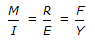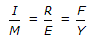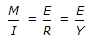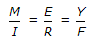# Civil Engineering - Theory of Structures

Exercise : Theory of Structures - Section 3
1.
If a concrete column 200 x 200 mm in cross-section is reinforced with four steel bars of 1200 mm2 total cross-sectional area. Calculate the safe load for the column if permissible stress in concrete is 5 N/mm2 and Es is 15 Ec
264 MN
274 MN
284 MN
294 MN
None of these.
Explanation:
No answer description is available. Let's discuss.

2.
If normal stresses due to longitudinal and transverse loads on a bar are σ1 and σ2 respectively, the normal component of the stress on an inclined plane θ° to the longitudinal load, is
σ1 sin θ x σ2 cos θ
σ1 sin θ2 + σ2 cos2 θ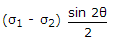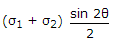Explanation:
No answer description is available. Let's discuss.

3.
A shaft subjected to a bending moment M and a torque T, experiences
maximum bending stress =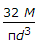maximum shear stress =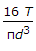both (a) and (b)
neither (a) nor (b)
Explanation:
No answer description is available. Let's discuss.

4.
In the truss shown in given figure the force in member DC is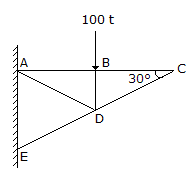100 t compressive
100 t tensile
zero
indeterminate